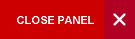#### Online Support## A Prediction Equation for the Estimation of Cardiorespiratory Fitness Using an Elliptical Motion Trainer

Journal Authors:
DOI:
10.7727/wimj.2012.194
Pages:
114–7

ABSTRACT

Objective: In the United States of America, 6.2 million individuals are using elliptical motion trainers in fitness centres. However, graded exercise test protocols to estimate peak oxygen consumption (VO2peak) using elliptical motion trainers have not been developed for the general population.

Methods: Fifty-nine subjects (mean age: 23.5 ± 4.1 years) were randomly divided into a validation (VAL: n = 39) or cross-validation (XVAL: n = 20) group. Peak oxygen consumption (ml⋅kg-1⋅min-1) was measured via indirect calorimetry on an elliptical motion trainer for both groups. Subjects exercised at 150 strides⋅min-1 against a resistance of four and a crossramp of 8%. The resistance was increased every two minutes by two units until exhaustion. For the VAL group, a stepwise regression analysis was used to predict VO2peak from resistance, maximal heart rate (HRmax), body mass index (BMI), height and gender (female = 0, male = 1).

Results: The prediction equation derived from this study was VO2peak (ml·kg-1.min-1) = 187.39403 + 12.97271 (gender) - 1.45311 (height) - 1.21604 (BMI) - 0.19613 (HRmax) + 1.57093 (resistance) (R2 = 0.76, SEE = 4.47, p < 0.05). Using this equation, the predicted VO2peak of the XVAL group was 45.18 ± 6.42 ml·kg-1⋅min-1, while the measured VO2peak was 43.55 ± 6.23 ml·kg-1⋅min-1

Conclusion: No significant difference was found between the measured and predicted VO2peak in the XVAL group. Therefore, it appears this protocol and equation will allow individuals to accurately
estimate their VO2peak without using direct calorimetry. However, future studies should investigate the validity of this protocol with diverse populations.

Accepted:
31 Aug, 2012
PDF Attachment:dr_brown_wimj_february.pdf
e-Published: 16 May, 2013
Top of Page